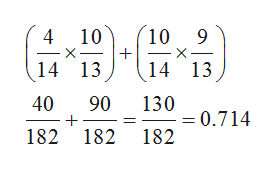involving the selection of two apples from a bag of red and yellow apples without replacement. Assume that the bag has a total of 14 apples: 10 red and 4 yellow.(1) What is the probability that the second apple you pick is red? (2) What is the probability that you pick at least one red apple?

Question

involving the selection of two apples from a bag of red and yellow apples without replacement. Assume that the bag has a total of 14 apples: 10 red and 4 yellow.

(1) What is the probability that the second apple you pick is red?

(2) What is the probability that you pick at least one red apple?

Step 1

According to the given information:

Number of red balls=10

Number of yellow balls=4

Total number of balls = 14

Step 2

In part (1) it is needed to calculate the probability that the second apple picked is red:

So, outcomes for second apple is red are:

Outcome 1...help_outlineImage Transcriptionclose10 9 4 10 14 13 14 13 40 90 130 =0.714 182 182 182 fullscreen

Want to see the full answer?

See Solution

Want to see this answer and more?

Our solutions are written by experts, many with advanced degrees, and available 24/7

See Solution
Tagged in

Math